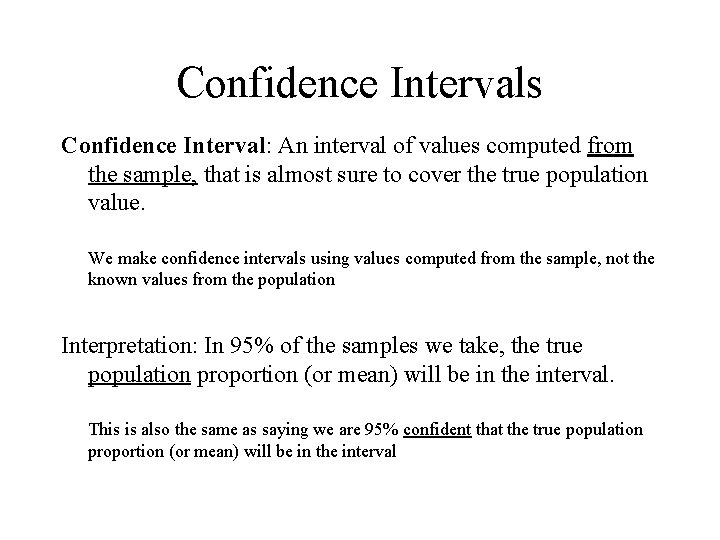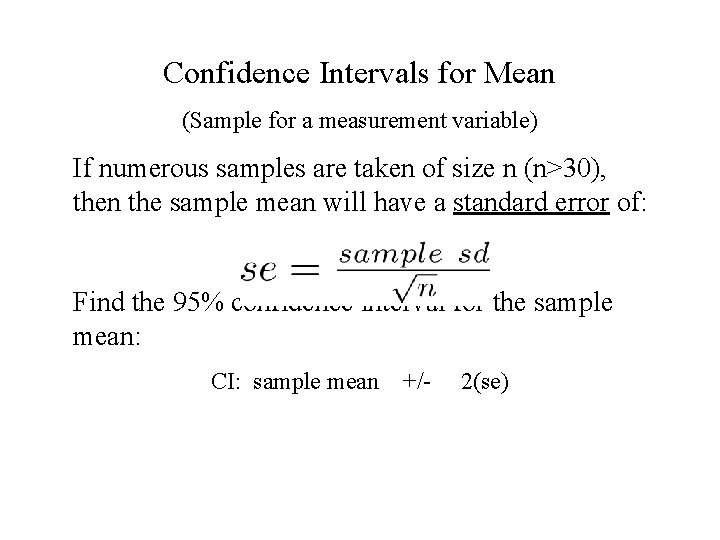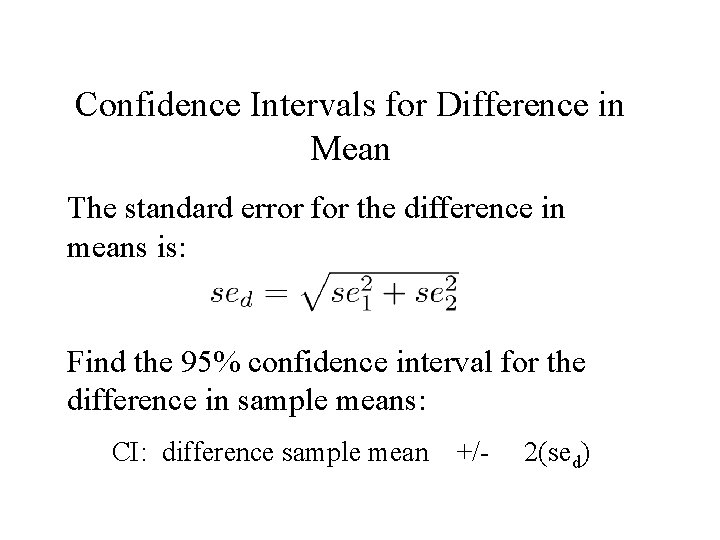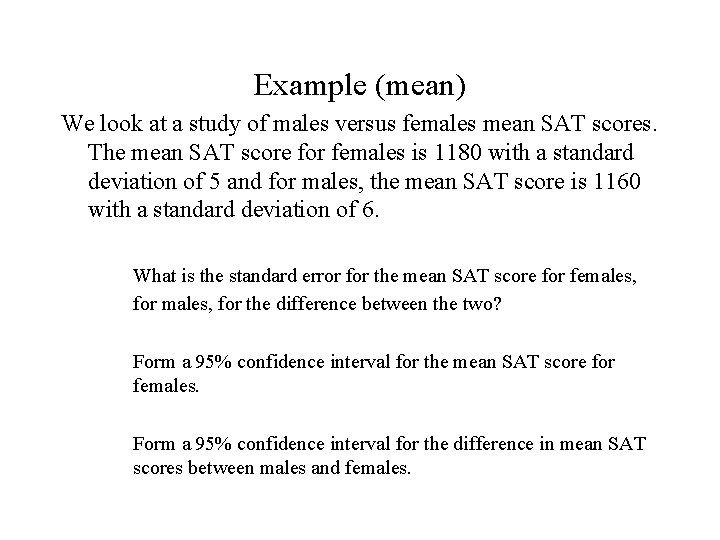# Confidence Intervals Confidence Interval An interval of values

• Slides: 7Confidence Intervals Confidence Interval: An interval of values computed from the sample, that is almost sure to cover the true population value. We make confidence intervals using values computed from the sample, not the known values from the population Interpretation: In 95% of the samples we take, the true population proportion (or mean) will be in the interval. This is also the same as saying we are 95% confident that the true population proportion (or mean) will be in the intervalHow do we compute the intervals? We know that in 95% of the samples, the true population proportion(or mean) will fall within in 2 standard errors of the sample mean. Where does the 2 come from: For a bell curve 95% of the data will be between +/- 1. 96 standard deviations. What is the standard error: This is not the standard deviation of the sample, it is the standard deviation of the sample proportion (or mean)Confidence Intervals for Proportions (Sample for a categorical variable) If numerous samples are taken of size n (n>30), then the sample proportion will have a standard error of: Where p is the sample proportion Find the 95% confidence interval for the sample proportion: CI: sample proportion +/- 2(se)Example (Proportion) A study was done on the proportion of males and females who participate in athletics. 480 females and 530 males participated in the study, where 170 females participated in athletics and 208 males. What is the sample of females participating in athletics? What is the standard error of the sample proportion of athletes? Construct a 95% confidence interval for the population proportion of females that would participate in athletics.Confidence Intervals for Mean (Sample for a measurement variable) If numerous samples are taken of size n (n>30), then the sample mean will have a standard error of: Find the 95% confidence interval for the sample mean: CI: sample mean +/- 2(se)Confidence Intervals for Difference in Mean The standard error for the difference in means is: Find the 95% confidence interval for the difference in sample means: CI: difference sample mean +/- 2(sed)Example (mean) We look at a study of males versus females mean SAT scores. The mean SAT score for females is 1180 with a standard deviation of 5 and for males, the mean SAT score is 1160 with a standard deviation of 6. What is the standard error for the mean SAT score for females, for the difference between the two? Form a 95% confidence interval for the mean SAT score for females. Form a 95% confidence interval for the difference in mean SAT scores between males and females.